### Home > PC > Chapter 8 > Lesson 8.1.2 > Problem8-25

8-25.
1. Given the function f(x) =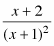. Homework Help ✎

1. Use your calculator to create a table for f(x). As x → ∞, describe what happens to f(x).

2. Describe how these table values are reflected in the graph of f(x).

3. Make a table for f(x) when −3 ≤ x ≤ 1 using increments of 0.2. Use your table values to evaluate the following limits: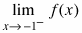and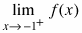.

4. What happens in the table at x = −1? How is this reflected in the graph?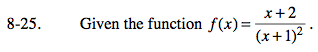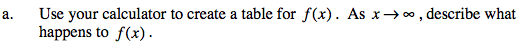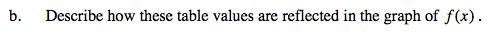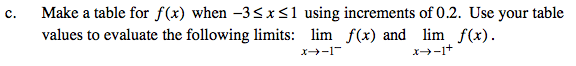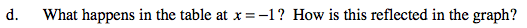Complete the table in the eTool below for part (a).
Click the link at right for the full version of the eTool: PCT 8-25 HW eTool